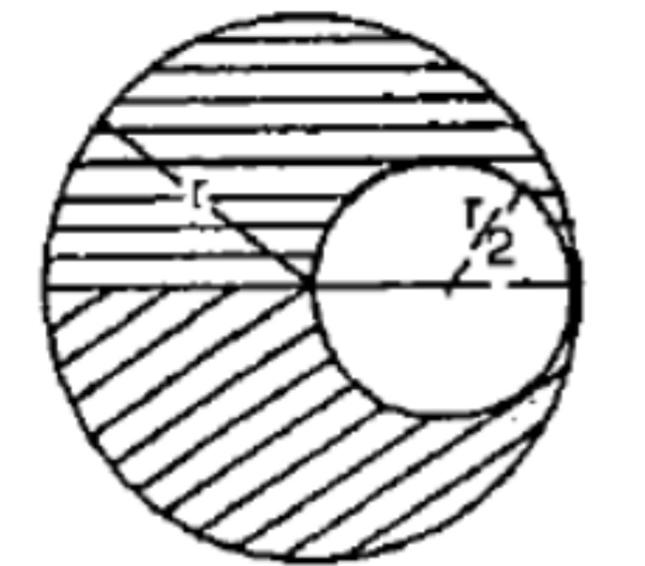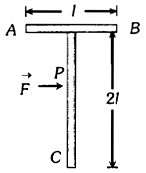A circular disc of radius r has a uniform thickness.  A circular hole of diameter equal to the radius of the disc has been cut out as shown.  The centre of gravity of the remaining disc lies on the diameter of the disc at a distance x to the left of the centre of the original disc.  The value of x is(1) r                              (2) $\frac{r}{2}$

(3) $\frac{r}{4}$                           (4) $\frac{r}{6}$

High Yielding Test Series + Question Bank - NEET 2020

Difficulty Level:

A 'T' shaped object with dimensions shown in the figure, is lying on a smooth floor. A force '$\stackrel{\to }{\mathrm{F}}$' is applied at the point P parallel to AB, such that the object has only the translational motion without rotation. Find the location of P with respect to C1.  $\frac{4}{3}l$

2.  I

3.  $\frac{2}{3}l$

4.  $\frac{3}{2}l$

Concept Questions :-

Center of mass
High Yielding Test Series + Question Bank - NEET 2020

Difficulty Level:

A wheel is rotating about an axis through its centre at 720 r.p.m. It is acted on by a constant torque opposing its motion for 8 seconds to bring it to rest finally. The value of torque is Nm is (given l = $\frac{24}{\mathrm{\pi }}$ kg ${\mathrm{m}}^{2}$)

1. 48

2. 72

3. 96

4. 120

Concept Questions :-

Torque
High Yielding Test Series + Question Bank - NEET 2020

Difficulty Level:

Concept Questions :-

Rolling motion
High Yielding Test Series + Question Bank - NEET 2020

Difficulty Level:

Assertion : The speed of whirlwind in a tornado is alarmingly high.

Reason    : If no external torque acts on a body, its angular velocity remains conserved.

(1) If both assertion and reason are true and reason is the correct explanation of assertion.

(2) If both assertion and reason are true but reason is not the correct explanation of assertion.

(3) If assertion is true but reason is false.

(4) If both assertion and reason are false.

Concept Questions :-

Angular momentum
High Yielding Test Series + Question Bank - NEET 2020

Difficulty Level:

A circular disc A of radius r is made from an iron plate of thickness t and another circular disc B of radius 2r and thickness $\frac{\mathrm{t}}{4}$. The relation between moments of inertia ${\mathrm{I}}_{\mathrm{A}}$ and ${\mathrm{I}}_{\mathrm{B}}$ is

1.

2.

3.

4.  Depends on the actual values of t and r

Concept Questions :-

Moment of inertia
High Yielding Test Series + Question Bank - NEET 2020

Difficulty Level:

A stationary bomb explodes into two parts of masses 3kg and 1kg. The total KE of the two parts after explosion is 2400 J. The KE of smaller part is

1. 600 J

2. 1800 J

3. 1200 J

4. 2160 J

Concept Questions :-

Linear momentum
High Yielding Test Series + Question Bank - NEET 2020

Difficulty Level:

Two balls of equal masses have a head-on collision with speed 6 m/s each. If the coefficient of restitution is 1/3, the speed of each ball after impact will be

1. 18 m/s

2. 2 m/s

3. 6 m/s

4. data insufficient

Concept Questions :-

Linear momentum
High Yielding Test Series + Question Bank - NEET 2020

Difficulty Level:

A wheel is rotating 900 rpm about its axis. When power is cut off it comes to rest in 1 min. The angular retardation in rad/s2 is

1. $\mathrm{\pi }/2$

2. $\mathrm{\pi }/4$

3. $\mathrm{\pi }/6$

4. $\mathrm{\pi }/8$

Concept Questions :-

Rotation motion : Introduction
High Yielding Test Series + Question Bank - NEET 2020

Difficulty Level:

A particle of mass 2 kg located at the position  m has a velocity of 2 m/s. Its angular momentum about z-axis in kg-${\mathrm{m}}^{2}/\mathrm{s}$ is :

1. +4

2. +8

3. -4

4. -8

Concept Questions :-

Angular momentum